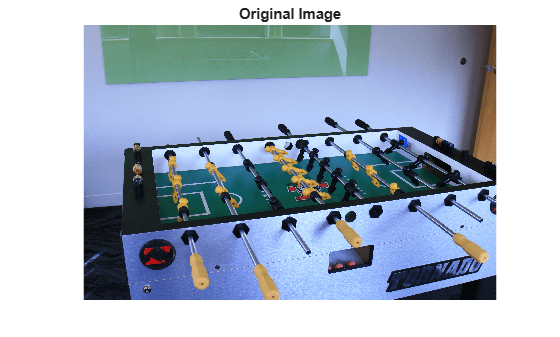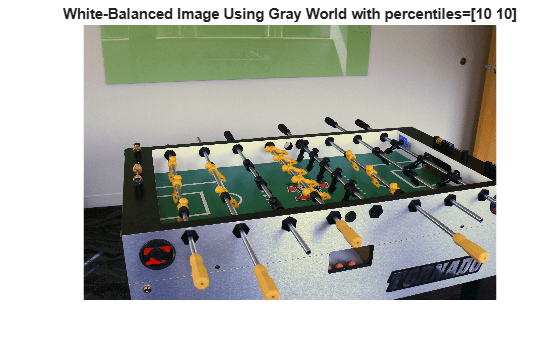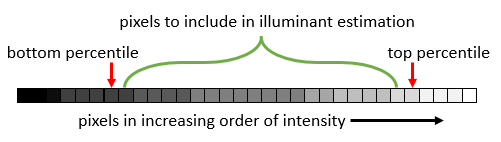illumgray

Estimate illuminant using gray world algorithm

Description

illuminant = illumgray(A) estimates the illumination of the scene in RGB image A by assuming that the average color of the scene is gray.

example

illuminant = illumgray(A,percentile) estimates the illumination, excluding the specified bottom and top percentiles of pixel values.

illuminant = illumgray(___,Name,Value) estimates the illumination using name-value pairs to control additional options.

Examples

collapse all

Open an image and display it. Specify an optional magnification to shrink the size of the displayed image.

figure
imshow(A,'InitialMagnification',25)
title('Original Image')The gray world algorithm assumes that the RGB values are linear. However, the JPEG file format saves images in the gamma-corrected sRGB color space. Undo the gamma correction by using the rgb2lin function.

A_lin = rgb2lin(A);

Estimate the scene illumination, excluding the top and bottom 10% of pixels. Because the input image has been linearized, illumgray returns the illuminant in the linear RGB color space.

percentiles = 10;
illuminant = illumgray(A_lin,percentiles)
illuminant = 1×3

0.2206    0.2985    0.5219

The third coefficient of illuminant is the largest, which is consistent with the blue tint of the image.

Correct colors by providing the estimated illuminant to the chromadapt function.

To display the white-balanced image correctly on the screen, apply gamma correction by using the lin2rgb function.

B = lin2rgb(B_lin);

Display the corrected image, setting the optional magnification.

figure
imshow(B,'InitialMagnification',25)
title(['White-Balanced Image Using Gray World with percentiles=[' ...
num2str(percentiles) ' ' num2str(percentiles) ']'])Input Arguments

collapse all

RGB image, specified as an m-by-n-by-3 numeric array.

Data Types: single | double | uint8 | uint16

Percentile of pixels to exclude from the illuminant estimation, specified as a numeric scalar or 2-element numeric vector. Excluding pixels helps prevent overexposed and underexposed pixels from skewing the estimation.

• If percentile is a scalar, the same value is used for both the bottom percentile and the top percentile. In this case, percentile must be in the range [0, 50] so that the sum of the bottom and top percentiles does not exceed 100.

• If percentile is a 2-element vector, the first element is the bottom percentile and the second element is the top percentile. Both percentiles must be in the range [0, 100) and their sum cannot exceed 100.

The following image indicates the range of pixels that are included in the illuminant estimation. The selection is separate for each color channel.Data Types: single | double | int8 | int16 | int32 | int64 | uint8 | uint16 | uint32 | uint64

Name-Value Arguments

Specify optional comma-separated pairs of Name,Value arguments. Name is the argument name and Value is the corresponding value. Name must appear inside quotes. You can specify several name and value pair arguments in any order as Name1,Value1,...,NameN,ValueN.

Example: illuminant = illumgray(I,'Mask',m) estimates the scene illuminant using a subset of pixels in image I, selected according to a binary mask, m.

Image mask, specified as the comma-separated pair consisting of 'Mask' and an m-by-n logical or numeric array. The mask indicates which pixels of the input image A to use when estimating the illuminant. The computation excludes pixels in A that correspond to a mask value of 0. By default, the mask has all 1s, and all pixels in A are included in the estimation.

Data Types: single | double | int8 | int16 | int32 | int64 | uint8 | uint16 | uint32 | uint64 | logical

Type of vector norm (p-norm), specified as the comma-separated pair consisting of 'Norm' and a positive numeric scalar. The p-norm affects the calculation of the average RGB value in the input image A. The p-norm is defined as sum(abs(x)p) ^ (1/p).

Data Types: single | double | int8 | int16 | int32 | int64 | uint8 | uint16 | uint32 | uint64

Output Arguments

collapse all

Estimate of scene illumination, returned as a 3-element numeric row vector. The three elements correspond to the red, green, and blue values of the illuminant.

Data Types: double

Tips

• The gray world algorithm assumes uniform illumination and linear RGB values. If you are working with nonlinear sRGB or Adobe RGB images, use the rgb2lin function to undo the gamma correction before using illumgray. Also, make sure to convert the chromatically adapted image back to sRGB by using the lin2rgb function.

• When you specify Mask, the bottom percentile and top percentile apply to the masked image.

• You can adjust the color balance of the image to remove the scene illumination by using the chromadapt function.

 Ebner, Marc. "The Gray World Assumption." Color Constancy. Chichester, West Sussex: John Wiley & Sons, 2007.